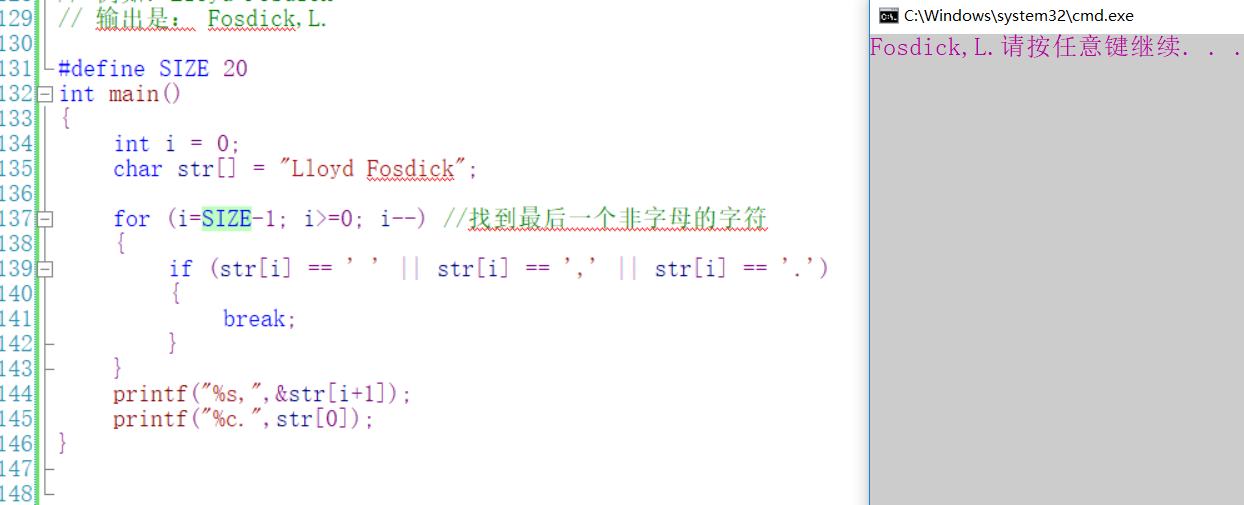c语言编写一个程序，根据用户输入英文名和姓先显示姓氏，其后跟一个逗号，然后显示名的首字母 10C

c语言编写一个程序，根据用户输入英文名和姓先显示姓氏，其后跟一个逗号，然后显示名的首字母。

10个回答

# include

int main()
{
char ch;
char chf;
char chl;
int i=0, j=0, k=0;
//录入字符串
while(scanf("%c", &ch) && ch!='\n')
{
chl[i]=ch;
i++;
}
//求前面的空格
for (int c=0;c if (chl[c]>=65 && chl[c]<=90)
break;

``````//去空格
for (int d=0;d<c;d++)
for (int e=c-1;e<i;e++)
chl[e-1]=chl[e];
i=i-c;

//找姓的首字母地址
for (j=1;j<i;j++)
if (chl[j]>=65 && chl[j]<=90)
break;

//录入姓
while (j<i)
{
chf[k]=chl[j];
k++;
j++;
}
//输出
for (int a=0; a<k; a++)
{
printf ("%c", chf[a]);
if (chf[a+1]==' ')
break;
}

printf (",");
printf ("%c",chl);
return 0;
``````

}

``````
``````2 年多之前 回复u011029018 如果是c语言，那就遍历字符串找到空格的位置，然后进行字符串的切片操作，如果是c++，直接有函数split进行操作。 在分割好后，先输出后面的字符串，再输出“,”，最后再输出字符串的第一个字母。
2 年多之前 回复whyyouhitme_ 回复GHH120338: 老哥，请注意题目。说是有可能会加空格。也就是，也有可能不加空格。所以我是按大写字母区分的。
2 年多之前 回复u011029018 如果是c语言，那就遍历字符串找到空格的位置，然后进行字符串的切片操作，如果是c++，直接有函数split进行操作。 在分割好后，先输出后面的字符串，再输出“,”，最后再输出字符串的第一个字母。
2 年多之前 回复2 年多之前 回复2 年多之前 回复whyyouhitme_ 回复Siege-lion: 老哥。如果用户的输入里没有空格呢？
2 年多之前 回复
``````  我的想法是:按老外的习惯，他们的首字母会大写，弄俩字符串，分别记录名和姓，遇到大写，就是65到106开始，把名输到名的字符串里面，遇到第二个大写输到姓的字符串里面，然后输出。
``````

``````#define SIZE 20
int main()
{
int i = 0;
char str[] = "Lloyd Fosdick";

for (i=SIZE-1; i>=0; i--) //找到最后一个非字母的字符
{
if (str[i] == ' ' || str[i] == ',' || str[i] == '.')
{
break;
}
}
printf("%s,",&str[i+1]);
printf("%c.",str);
}

``````/*去除字符串右边空格*/

void StrRTrim(char *pStr)

{

char *pTmp = pStr+strlen(pStr)-1;

``````while (*pTmp == ' ')
{
*pTmp = '\0';
pTmp--;
}
``````

}

/*去除字符串左边空格*/

void StrLTrim(char *pStr)

{

char *pTmp = pStr;

``````while (*pTmp == ' ')
{
pTmp++;
}
while(*pTmp != '\0')
{
*pStr = *pTmp;
pStr++;
pTmp++;
}
*pStr = '\0';
``````

}

int StrName(char *pStr, int nLen)
{
int i = 0;

``````for (i=nLen-1; i>=0; i--) //找到最后一个非字母的字符
{
if (pStr[i] == ' ')
{
break;
}
}
printf("%s,",pStr + i);
printf("%c.", *pStr);
return 0;
``````

}

void main()
{
char str[] = " Lloyd Fosdick ";
StrLTrim(str);
StrRTrim(str);
StrName(str, strlen(str));
}

#include
#include
int main(int argc, char **argv)
{
char s_name;
char f_name;
char l_name;
int i=0;

``````    printf("please enter your name!\n");
fgets(s_name, 256, stdin);
printf("%s\n",s_name);
strncpy(f_name, s_name, 1);
printf("%s\n", f_name);
for (i=1; s_name[i] != '\0'; i++)
{
if (s_name[i] >= 'A' && s_name[i] <= 'Z')
{
break;
}
}
strncpy(l_name, s_name+i, strlen(s_name)-i-1);

printf("%s,%s\n", l_name, f_name);

return 0;
``````

}

``````
``````Vist_1 复制的有问题，前两行是#include <stdio.h>和#include <string.h>
2 年多之前 回复C语言，输入星期首字母输出星期名，请问这小程序哪里错了，

C语言文件简单加密与解密

C#控制台程序，怎么把用户输入的姓名和成绩中的前三名（考虑并列情况）输出

c语言 给国家的名称按字典顺序排序

c语言简单学生查询问题

![输入q类型不一样，出问题](https://img-ask.csdn.net/upload/201706/19/1497834160_477104.png) ``` #include <stdio.h> #include <string.h> #define NUMBER 5 //学生人数 //1).编写函数average，对n个学生的成绩score，计算平均分数，并返回其值。 int arevage(int score[]) { int total=0; int i=0; for (i=0;i<NUMBER;i++) { total+=score[i]; //总学生成绩 } return total/NUMBER; //得出平均值 } //2).编写函数grade，对n个学生的成绩score，分别统计及格人数c1和不及格的人数c2，并输出。 int grade(int score[]) { int c1=0;//及格人数 int c2=0;//不及格人数 int i=0; for (i=0;i<NUMBER;i++) { if(score[i]>60) c1++; else c2++; } printf ("及格人数为： %d 人\n",c1); printf ("不及格人数为： %d 人\n",c2); } //3).编写函数max，求n个学生的成绩score的最高分，并返回其值。 int max(int score[]) { int i=0,j=0; int _max=0; for (i=0;i<NUMBER-1;i++) { for(j=i+1;j<NUMBER;j++) { if(score[i]>score[j]) { _max=score[i]; score[i]=score[j]; score[j]=_max; } } } return score[i]; } //4)、编写函数xx，对n个学生的成绩score进行排序，并输出。 int xx(int score[]) { int i=0,j=0; int _max=0; for (i=0;i<NUMBER-1;i++) { for(j=i+1;j<NUMBER;j++) { if(score[i]>score[j]) { _max=score[i]; score[i]=score[j]; score[j]=_max; } } } return 0; } /*5.)编写主函数main，通过键盘输入用户的选择步骤完成相应功能，用户输入1时调用average统计平均分，输入2时调用grade统计及格或不及格人数，输入3时调用max找最大值，输入4时调用xx对成绩排序，输入0时返回。 程序要求：合适繁荣提示语 */ int menu(int _getNumber) { int new; int i=(int)_getNumber; int studentScore[NUMBER]={10,70,61,90,100}; if(i=='q'||i=='Q') { exit(1); } while(i!=1&&i!=2&&i!=3&&i!=4) { printf ("\$error :输入有误请重新输入！\a\n"); scanf("%d",&i); printf ("hello word !\n"); //scanf("%d",&i); } while(i==1||i==2||i==3||i==4) { //计算机平均分 if (i==1) { printf ("平均成绩是：%d\n",arevage(studentScore)); //continue; } //统计合格与不合格的人数 if (i==2) { grade(studentScore); //continue; } //最高成绩 if (i==3) { printf ("最高的成绩为： %d\n",max(studentScore)); } //从小到大排序 if (i==4) { xx(studentScore); for (i=0;i<NUMBER;i++) { printf ("从小到大排序后为：sutdent[%d] = %d\n",i,studentScore[i]); } } printf ("\$请再输入查询代号:"); scanf("%d",&i); if(i!=1&&i!=2&&i!=3&&i!=4) { printf ("\$error :输入有误请重新输入！\a\n"); if(scanf("%d",&i)); } if(i=='q'&&i=='Q') { break; } //printf("i = %d",i); if(i!=1&&i!=2&&i!=3&&i!=4) { printf ("\$error :输入有误请重新输入！\a\n"); scanf("%d",&i); } } } int main(int argc,char *argv[]) { int getNumber=0; printf ("***************************************************\n\n"); printf (" 输入 【1】————————统计学生成绩\n"); printf (" 输入 【2】————————分别统计学生成绩及格人数和不及格的人数\n "); printf ("输入 【3】————————统计学生的最高成绩\n "); printf ("输入 【4】————————对学生成绩进行排序\n "); printf ("输入 【q】或者【Q】————————退出程序\n "); printf ("\$你输入查询代号:"); scanf("%d",&getNumber); menu(getNumber); return 0; } ``` 主要是：让用户输入q,或者Q退出程序，输入1-4，输入其他报错，让用户重新输入 我调试了下是，当输入英文时，但scanf要求输入 整数类型，类型不一，里面的值不是本身的值，如何解决这个问题？

#include<stdio.h> int main() { char s; char a; printf("Please enter a string and a character: "); scanf("%s%c",s,&a); int i; int j=0; for(i=0;s[i]!='\0';i++) { if(s[i]!=a) { s[j++]=s[i]; } } s[j]='\0'; printf("%s",s); return 0; }

Problem Description 当寒月还在读大一的时候，他在一本武林秘籍中（据后来考证，估计是计算机基础，狂汗-ing），发现了神奇的二进制数。 如果一个正整数m表示成二进制，它的位数为n（不包含前导0），寒月称它为一个n二进制数。所有的n二进制数中，1的总个数被称为n对应的月之数。 例如，3二进制数总共有4个，分别是4（100）、5（101）、6（110）、7（111），他们中1的个数一共是1＋2＋2＋3=8，所以3对应的月之数就是8。 Input 给你一个整数T，表示输入数据的组数，接下来有T行，每行包含一个正整数 n（1<=n<=20）。 Output 对于每个n ，在一行内输出n对应的月之数。 Sample Input 3 1 2 3 Sample Output 1 3 8

C语言编程 作业求助 求大神来帮忙

1、写一个函数计算1+4+9+16+25+...+10000的值。 2、写一个函数，计算1-2+3-4+5-6+...-10000的值。 3、写一个函数，判断一个四位数是否符合如下条件：这个四位数的逆序排列所得的四位数是它自身的整数倍，如果符合，则返回1，不符合返回0。在主程序中调用这个函数，输出符合这个条件的所有的四位数。 4、写一个函数，输出如下格式的图形，要求输出的行数由参数决定，在主函数中输入行数，调用子函数，输出相应行数的图形： * *** ****** ******** ****** *** * 5、如果有一个正整数从左、右来读都是一样的，则称为回文式数(简称回数)；比如101，32123，999都是回数。数学中有名的“回数猜想”之迷，至今未解决。 回数猜想： 任取一个数，再把它倒过来，并把这两个数相加，然后把这个和数再倒过来，与原和数相加，重复此过程，一定能获得一个回数。 例： 68 倒过来是 86， 68+86= 154，154+541= 605，605+506=1111 (回数) 编程，输入任意整数，按上述方法产生一个回数，为简便起见，最多计算7步，看是否能得到一个回数。 要求： 1）主函数中接收键盘数据，必须用scanf(“%ld”,&变量名)接收整型变量，显示该数与其倒数的和，输出每一步计算步骤。 2）子函数1，计算该数的倒数。 3）子函数2，验证和是否为回数。 4）如果经过7次以内的计算得出的数是回数，则主函数打印“经过n次计算，得到回数”，超过7次未能得到回数，显示，“经过n次计算，未得到回数”。

package test; import java.util.Scanner; public class LeapYear { public static void main(String[] args) { Scanner scan = new java.util.Scanner(System.in); System.out.println("请输入名字"); String name = scan.nextLine(); System.out.println("请输入电话"); String number = scan.nextLine(); scan.close(); } }

C语言指针冒泡排序问题

#include<stdio.h> #include<string.h> int main(){ void sort(char *name[],int n); char * alphabet[ ]={"Follow me","BASIC","Great Wall","FORTRAN","Computer design"}; int n = 5; sort(alphabet,n); for(int i=0;i<5;i++) printf("%s\n",alphabet[i]); return 0; } void sort(char *name[],int n){ int i=0,j=0; char *temp; for(;i<=4;i++){ for(;j<=n-j-1;j++){ if(strcmp(name[j],name[j+1])>0){ temp = name[j]; name[j] = name[j+1]; name[j+1] = temp; } } } } 代码如上，想要通过ASCII码来比较alphabet中的字符串，并按照英文字母的顺序输出。函数SORT重用的冒泡排序，但输出的结果里只有第一位和第二位正确调换了位置，不知道问题出在哪里？请指教！万分感谢！！！

“第M大数”是指从小到大的第M大，具体怎么计算采用的是C语言的程序的编写的技术的办法

Problem Description 　　Google的面试题向来以古怪闻名,延续自技术公司用逻辑题测试求职者的古老传统.现在我们来看看下面这题: 　　面试官在房间的白板上写下6个数字: 　　　　10,9,60,90,70,66 　　现在的问题是，接下来该出现什么数字？ 　　想不出来了吧?不要再从数学的角度想了,把这些数字用正常的英文拼写出来: 　　　　ten(10) 　　　　nine(9) 　　　　sixty(60) 　　　　ninety(90) 　　　　seventy(70) 　　　　sixty-six(66) 　　我们可以惊奇的发现这些数字都是按字母的多少排序的!再仔细一看:ten(10)不是唯一一个可以用3个字母拼出的数字,还有one(1),two(2),six(6);nine(9)也不是唯一一个用4个字母拼出的数字,还有zero(0),four(4)和five(5).而题目中的数字,每一个都是用给定长度的字母拼写出来的数字里最大的一个！ 　　现在我们回到原题:接下去该是哪个数字呢? 　　我们注意到，66对应的字母长度为8（特别提醒：连接符不算在内），不管之后跟着哪个数，它都应该有9个字母，而且应该是9个字母拼出的数字里最大的。仔细找一下，你可能就会得出ninety-six(96)。不可能是100以上的数字，因为它会以one hundred开头,这已经有10个字母了。 　　对于Google面试官来说，96只不过是可以接受的答案之一，另一个更好的回答是: 　　100000000000000000000000000000000000000000000000000000000000000000000000000000000000000000000000000000 　　也就是10的101次方，即：ten googol（有关Googol的资料可以在wiki中了解）。据说当年Google这个名字的创建也是由googol演化过来的（江湖传说肖恩拼写时老爱出错，本来想注册googol或者googolplex，结果由于手误就注册了google）。 　　好了，当你解出了这道难题，面试官的下一道题目接踵而至——给你两个正整数N和M，要求你输出由N个字母组成的第M大数(我们只考虑0~99和googol级别的数字)。 注意：这里所说的“第M大数”是指从小到大的第M大，具体参见Sample Input 输入数据第一行有一个数字T，代表有T组数据。 每组数字由两个正整数N和M组成。 [Technical Specification] 1<=T<=100 3<=N<=9 1<=M<=100 Output 首先输出case数(见sample)，接着输出由N个字母组成的第M大数，如果没有，则输出-1。 Sample Input 6 3 1 3 2 4 1 4 2 5 1 9 100 Sample Output Case #1: 1 Case #2: 2 Case #3: 0 Case #4: 4 Case #5: 3 Case #6: -1

#include<stdio.h> #include<string.h> int main() { char s1; int a; int b; char s2; int n; int i; char temp1; char temp2; char temp3; char temp4; while(scanf("%d",&n)!=EOF) { for(i=0;i<n;i++) { scanf("%d",&a[i]); scanf("%s",&s1[i]); scanf("%d",&b[i]); scanf("%s",&s2[i]); } for(i=0;i<n;i++) { int k=i+1; while(k<n) { int j; for(j=0;j<strlen(s1[k])||j<strlen(s1[i]);j++) { if((s1[i][j]-s1[k][j])>0) { strcpy(temp3[k],s1[i]); strcpy(temp4[i],s1[k]); strcpy(s1[k],temp3[k]); strcpy(s1[i],temp4[i]); strcpy(temp1[k],s2[i]); strcpy(temp2[i],s2[k]); strcpy(s2[k],temp1[k]); strcpy(s2[i],temp2[i]); int t=b[i]; b[i]=b[k]; b[k]=t; break; } else if((s1[i][j]-s1[k][j])<0) break; } k++; } } int k=1; for(i=0;i<n;i++) { if(s1[i]=='C'&&s1[i]=='h'&&s1[i]=='i'&&s1[i]=='n'&&s1[i]=='a') continue; printf("%d %s %d %s\n",k,s1[i],b[i],s2[i]); k++; } for(i=0;i<n;i++) { if(s1[i]=='C'&&s1[i]=='h'&&s1[i]=='i'&&s1[i]=='n'&&s1[i]=='a') { printf("%d %s %d %s\n",k,s1[i],b[i],s2[i]); k++; } } } return 0; } 按照国家名字的英文字母顺序来出场。请编写一个计算机程序实现国家名字的排序（升序），中国（China）在最后一个 输入描述： 多组输入，其中每组输入的第一行表示参加国家数 n（占一行），下面 n 行是国家的报名信息（序号、国家名称、参加人数、所属大洲） 输出描述： 按国家名字的字母序输出信息（东道主国家名字最后输出）。 样例输入: 5 1 France 123 Europe 2 Russia 254 Europe 3 Japan 235 Asia 4 Germany 185 Europe 5 China 560 Asia 样例输出: 1 France 123 Europe 4 Germany 185 Europe 3 Japan 235 Asia 2 Russia 254 Europe 5 China 560 Asia

loonggg读完需要3分钟速读仅需 1 分钟大家好，我是你们的校长。我之前讲过，这年头，只要肯动脑，肯行动，程序员凭借自己的技术，赚钱的方式还是有很多种的。仅仅靠在公司出卖自己的劳动时...

MySQL数据库面试题（2020最新版）

!大部分程序员只会写3年代码

HTTP与HTTPS的区别

《Oracle Java SE编程自学与面试指南》最佳学习路线图（2020最新版）

01、Java入门（Getting Started）;02、集成开发环境（IDE）;03、项目结构（Eclipse JavaProject）;04、类和对象（Classes and Objects）;05：词法结构（Lexical Structure）;06：数据类型和变量（Data Type and Variables）;07：运算符（Operators）;08：控制流程语句（Control Flow Statements）;